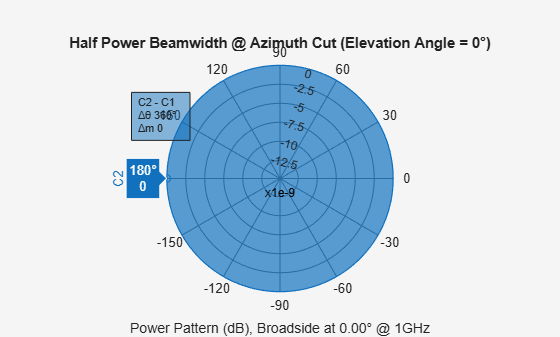beamwidth

Compute and display beamwidth of sensor element pattern

Description

example

beamwidth(element,freq) plots the 2-D power pattern (in dB) of the element for all azimuth angles at an elevation angle of zero degrees. The plot displays the half-power beamwidth (in degrees) at the frequency specified in freq (in Hz) and the angles (in degrees) in azimuth at which the magnitude of the power pattern decreases by 3 dB from the peak of the main beam.

beamwidth(element,freq,Name,Value) plots the beamwidth with the specified parameter Name set to the specified Value. You can specify additional name-value pair arguments in any order as (Name1,Value1,...,NameN,ValueN).

Example: beamwidth(element,1e9,'Cut','Elevation)

example

[bw,angles] = beamwidth(___) returns the angular beamwidth bw (in degrees). The function also returns the corresponding angle values (in degrees) that mark the beamwidth.

Examples

collapse all

Plot the beamwidth for an isotropic antenna at 1 GHz.

Create an isotropic antenna using a phased.IsotropicAntennaElement object.

antenna = phased.IsotropicAntennaElement('FrequencyRange',[800e6 1.2e9])
antenna =
phased.IsotropicAntennaElement with properties:

FrequencyRange: [800000000 1.2000e+09]
BackBaffled: false

Using the beamwidth function, plot the half-power (3 dB) beamwidth for the antenna. Use a frequency value of 1 GHz.

beamwidth(antenna, 1e9)ans = 360

Calculate the half-power beamwidth and angles of a cosine antenna element.

Create a phased.CosineAntennaElement object with the 'CosinePower' exponents set to 10.

myAnt = phased.CosineAntennaElement('CosinePower',[10 10])
myAnt =
phased.CosineAntennaElement with properties:

FrequencyRange: [0 1.0000e+20]
CosinePower: [10 10]

Compute the beamwidth and angles of the antenna element when it is operating at 1 GHz. Set 'dbDown' to 3 dB.

[BW,Ang] = beamwidth(myAnt,1e9,'dbDown',3)
BW = 29.9600
Ang = 1×2

-14.9800   14.9800

Input Arguments

collapse all

Antenna or microphone element, specified as a Phased Array System Toolbox System object.

Frequency used to calculate the beamwidth, specified as a scalar in Hz.

Example: 1e9

Data Types: double

Name-Value Arguments

Specify optional comma-separated pairs of Name,Value arguments. Name is the argument name and Value is the corresponding value. Name must appear inside quotes. You can specify several name and value pair arguments in any order as Name1,Value1,...,NameN,ValueN.

Example: beamwidth(element,1e9,'Cut','Azimuth','CutAngle',45) plots the beamwidth of the antenna element that is operating at a frequency of 1 GHz, with the slice direction set to 'Azimuth', and the cut angle set to 45 degrees.

The slice direction in azimuth-elevation space along which the beamwidth is computed, specified as the comma-separated pair consisting of 'Cut' and 'Azimuth' for the azimuth plane, and 'Cut' and 'Elevation' for the elevation plane.

Corresponding angle (in degrees) for the plane to get the required 2-D cut, specified as the comma-separated pair consisting of 'CutAngle' and a scalar. If 'Cut' is specified as 'Azimuth', then 'CutAngle' (Elevation) should lie between [−90, 90] degrees. If 'Cut' is specified as 'Elevation', then 'CutAngle' (Azimuth) should lie between [−180, 180] degrees.

Data Types: double

Power value (in dB) from the peak of the main lobe, specified as the comma-separated pair consisting of 'dBDown' and a positive scalar. The default value is 3 dB, which translates to half-power beamwidth. To calculate the first-null beamwidth, specify the 'dBDown' value as Inf.

Data Types: single | double | int8 | int16 | int32 | int64 | uint8 | uint16 | uint32 | uint64

Output Arguments

collapse all

Angular beamwidth of the sensor element, returned as a scalar in degrees.

Data Types: double

Angle values of the beamwidth, returned as a 1-by-2 vector. The two elements in the vector [amin, amax] define the beamwidth bw as amaxamin.